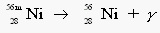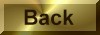Practice Problem 3

Predict the products of the following nuclear reactions:

(a)  electron emission by 14C                   (b)   positron emission by 8B

(c)  electron capture by 125I                    (d)   alpha emission by 210Rn

(e)  gamma-ray emission by 56mNi

Answer

We can predict the product of each reaction by writing an equation in which both mass number and charge are conserved:

(a)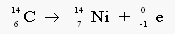(b)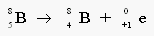(c)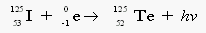(d)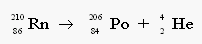(e)